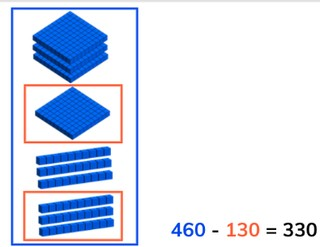Subtraction to 1,000 with multiples of ten

# Subtraction to 1,000 with multiples of ten

Subtract numbers to 1,000 with multiples of ten.

No account needed.8,000 schools use Gynzy92,000 teachers use Gynzy1,600,000 students use Gynzy

## General

Students learn to subtract numbers to 1,000 with multiples of ten, without crossing tens or hundreds.

## Standards

CCSS.Math.Content.2.NBT.B.7

## Learning objective

Students will be able to subtract multiples of ten up to 1,000.

## Introduction

Ask students to solve how many were taken away for each set of flags. You can first take away the hundreds, followed by the tens, and then the ones. Ask students to explain their strategy and to solve saying the steps out loud.

## Development

Start by reminding students that multiples of ten are numbers that end on a tens number. Show that a multiple of ten can also be a hundreds number that ends on a tens number. Then tell students that you can subtract by counting quantities. You first count how many there are in total, this is the first number in the subtraction problem (the minuend). You then count how many are taken away, this is the second number in the subtraction problem (the subtrahend). When you take the subtrahend away from the minuend you are left with the difference. Show this on the interactive whiteboard with the using the blocks, and practice a few subtraction problems. Show that this can be done with hundreds numbers, with tens numbers, and with multiples of ten. Check that students are able to subtract numbers from each other with the visual support of the blocks. Ask students to say or write the subtraction problem they are solving and to explain how they are subtracting. Then explain to students that you can subtract in different ways. You can use the number line to take the second number (the subtrahend) away from the first number (the minuend) to find the difference. You can also decide to split the subtrahend and take it away from the minuend in parts, so in hundreds and tens for example. You can also choose to subtract the second number from the first in one step. Emphasize that it is important to look at the subtraction problem first and then to determine which strategy you want to use to subtract. Each problem is different. Ask students to solve a few subtraction problems and ask them to explain their strategy. Then discuss how to solve a story problem with the class. Remind them of the steps of solving a story problem- start by determining what kind of math problem it is, then determine which numbers are important for the math problem. Say or write the math problem, and finally solve the math problem. After solving one story problem as a class, have students solve another story problem individually or in pairs.

Check that students are able to subtract numbers to 1,000 with multiples of ten by asking the following questions:
- What steps do you take to solve a story problem?
- How do you solve 940 - 730?

## Guided Practice

Students are given subtraction problems with visual support, as abstract problems, and story problems.

## Closing

Discuss with students that it is important to be able to subtract numbers to 1,000 with multiples of ten so that you can determine how many you have left with larger numbers. Ask them to solve the problems on the interactive whiteboard. Have students explain their strategy. Remind students that none of the strategies are 'better' but that different students and different problems require different strategies.

## Teaching tips

Support students who have difficulty subtracting multiples of ten by making use of a number line. That way they can visually see the jumps of numbers that they are taking away, and the subtraction is given context.

## Instruction materials

MAB-blocks or other manipulatives

### The online teaching platform for interactive whiteboards and displays in schools

• Save time building lessons

• Manage the classroom more efficiently

• Increase student engagement